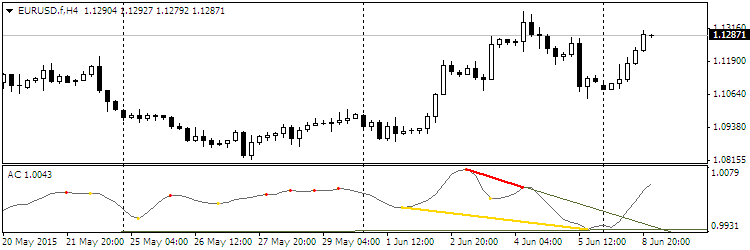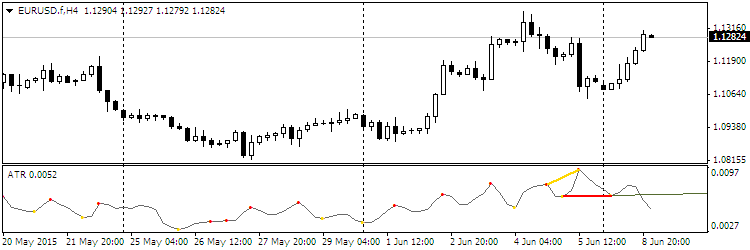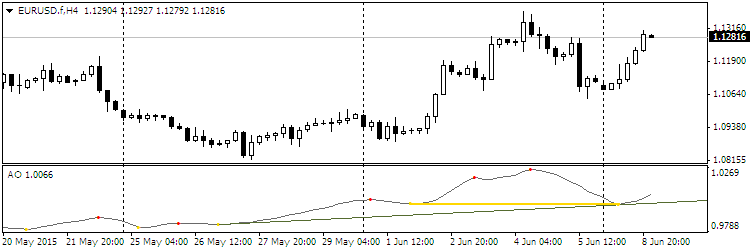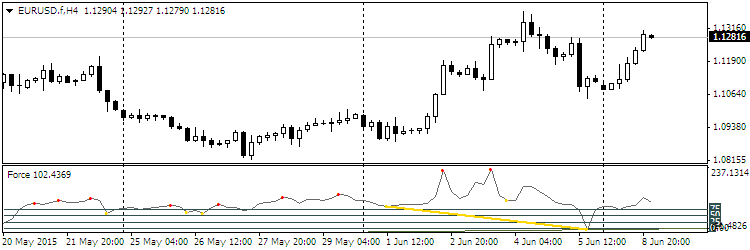# All about Oscillator & Histogram

This is a discussion on All about Oscillator & Histogram within the Trading tools forums, part of the Trading Forum category; Indicator_Strength These indicator have 3 Choice [ MA ; MACD ; Stochastic ] for displays in histogram form. The difference ...

 Tweet
1. ## All about Oscillator & Histogram

Indicator_Strength

These indicator have 3 Choice [ MA ; MACD ; Stochastic ] for displays in histogram form.

The difference between either :

1. MA_Power=true ~ a fast and slow Moving Average.

2. MACD_Power=true ~ main and signal lines of a MACD indicator.

3. Stochastic_Power=true ~ main and signal lines of a stochastic indicator.

Only one option can be choosen (default is [MA] Moving Average) at any one time, although another indicator can be opened for another option.

This indicator also displays average (strength) levels of difference values above and below the zero line. The feature can be disabled (Set_Levels=false).
This indicator is meant to gauge the power/strength behind a price move.Reply With Quote

2. divergencepetr_s7

Description:

To determine the divergence can select one of 30 oscillator.

Indicator:

• extreme peaks in the form of dots (red - the highs and yellow - a minimum);
• Divergence using segments connecting the extremes;
• support / resistance lines in the form of rays passing through the extremes;
• Channels in the form of rays.

Choosing the right oscillator is held in the first line of entering the number of the oscillator - parameter Osc. By default, the indicator RSI (number 29).

Code:
```
Selecting oscillators:

1. Accelerator
2. Accumulation / Distribution
3. Average Directional Movement Index
4. Average True Range
5. Awesome oscillator
6. Bears Power
7. Bulls Power
8. Commodity Channel Index
9. DeMarker
10. Force Index
11. Momentum
12. Money Flow Index
13. Moving Averages Convergence / Divergence
14. Moving Average of Oscillator
15. On Balance Volume
16. Relative Vigor Index
17. Standard Deviation
18. Stochastic Oscillator
19. Volume
20. Close
21. Open
22. High
23. Low
24. (H + L) / 2
25. (H + L + C) / 3
26. (H + L + C + C) / 4
27. (O + C + H + L) / 4
28. (O + C) / 2
29. Relative Strength Index
30. Larry Williams' Percent Range
31. ... Relative Strength Index

The list of parameters to adjust:

Osc = 29 - the number of the oscillator;  TH = true - bearish divergence;  TL = true - a bullish divergence;  trend = true - support / resistance lines;  convergen = true - hidden divergence;  Complect = 1 - the distinguishing index of the indicator on the chart;  BackSteph = 0 - number of steps backwards h;  BackStepl = 0 - number of steps back l;  BackStep = 0 - number of steps backward;  qSteps = 1 - the number of displayed steps, not more than three;  LevDPl = 5 - level points DeMark (2 = central bar will be above (below) the two bars on the left);  LevDPr = 1 - level points DeMark (2 = central bar will be above (below) the two bars on the right);  period = 8 - period for iADX, iATR, iBearsPower, iBullsPower, iCCI,  iDeMarker, iForce, iMomentum, iMFI, iRVI, iStdDev, iRSI, iRSI, iWPR;
ma_method = 0 - averaging method for iStochastic, iStdDev, iForce;  ma_shift = 0 - the average for the shift iStdDev;  applied_price = 4 - price type for iRSI, iRSI, iStdDev, iOBV, iOsMA,  iMACD, iMomentum, iForce, iCCIiBullsPower, iBearsPower, iADX;
mode = 0 - the index of the line for iStochastic, iRVI, iMACD, iADX;  fast_ema_period = 12 - the average for the period of rapid iOsMA, iMACD;  slow_ema_period = 26 - the period for slow average iOsMA, iMACD;  signal_period = 9 - the period of the signal line to iOsMA, iMACD;  Kperiod = 13 - K for the period of line iStochastic;  Dperiod = 5 - D line for the period iStochastic;  slowing = 3 - moderation iStochastic;  price_field = 0 - the type of prices for iStochastic;  showBars = 1000 - if = 0, the indicator is shown for the entire chart;  Trend_Down = true - the line resistance;  Trend_Up = true - line support;  TrendLine = true - support / resistance lines;  HandyColour = true;  Highline = Red - the color channel HL;  Lowline = DeepSkyBlue - color channel LL;  ChannelLine = false - channels in parallel trend lines;  Trend = 0 - 1 = only UpTrendLines, -1 = only DownTrendLines, 0 = all TrendLines;  Channel = false - channels;  Regression = true - channel linear regression, false - the standard deviation of the channel;  RayH = true - beam channel High;  RayL = true - beam channel Low;  ChannelH = Red - the color channel High;  ChannelL = DeepSkyBlue - color channel Low;  STD_widthH = 1.0 - sets the size of the deviation for the object CHANNEL High;  STD_widthL = 1.0 - sets the size of the deviation for the object CHANNEL Low;  comment = false - Displays comments;  code = 159 - Code for the Wingdings font symbol to denote the extrema;  BuyStop = false - levels BuyStop;  SellLimit = false - levels SellLimit;  SellStop = false - levels SellStop;  BuyLimit = false - levels BuyLimit.

Color oscillator and extreme points of customizable tab Colors.  When installing more than one indicator to the chart to change parameters Complect.    ```

Examples:Reply With Quote

3. MBMCD

Indicator as a demonstration based on the idea MaByMa can estimate the speed (lineCount = 1), acceleration (lineCount = 2) and other derivatives of the moving average or the price at the option ma = 1. The indicator looks like macd - and indeed convergence and divergence allow us ma information on the average rate of change of the price (rate of change). Compared with macd - indicator provides much more opportunities. You should be able to receive the following derivatives of 4 mA, and after small changes in the code and how many you want.

The fact that the receipt of a signal of trend reversal on the principle mabyma, much superior to signaling systems on the basis of MA crossing is well demonstrated in my publication entitled mabyma . By this I think that it is time to abandon the systems on the basis of convergence MA and move to more advanced systems based on MBMCD. That is replace MACD - MBMCD.

Default color chosen for use on a dark background.

Options:

Ma - Masha on which to build indicator. When ma = 1 the price.

mbm - Velocity convergence Masek - roughly average speeds.

lineCount - line betting the higher the number the more eign signal will be from 1 to 5 it.
When lineCount = 1 we get the speed, lineCount = 2 acceleration and so on. The parameter must be greater than 0 and less than or equal to 5.Reply With Quote

4. Awsome...

thanks for sharing

best regardsOriginally Posted by TsarIndicator_Strength

These indicator have 3 Choice [ MA ; MACD ; Stochastic ] for displays in histogram form.

The difference between either :

1. MA_Power=true ~ a fast and slow Moving Average.

2. MACD_Power=true ~ main and signal lines of a MACD indicator.

3. Stochastic_Power=true ~ main and signal lines of a stochastic indicator.

Only one option can be choosen (default is [MA] Moving Average) at any one time, although another indicator can be opened for another option.

This indicator also displays average (strength) levels of difference values above and below the zero line. The feature can be disabled (Set_Levels=false).
This indicator is meant to gauge the power/strength behind a price move.Reply With Quote

5. Strength indicator seems to be excellent in combination of all 3...

Have you tried before in live or demo trading? Any suggestions or results....Also does this repaint?

thanksReply With Quote

These OsMA [Oscillator of Moving Average] with Impulse & included AlertsReply With Quote

7. PricePercentRange - indicator for MetaTrader 4

This indicator can be used to estimate the direction of the trend in caswe we are using our trading system to decide concerning buy/sell trade for example (and when to open the trades).

The rules about how to use the indicator:

Price(%)Range is the indicator for the MetaTrader 4, which calculates the price movement based on percentage High (Highest) and Low (Lowest) price on 100 bars. The indicator will provide alerts when price changes direction.

This indicator is only appropriate for trading Trend.
If the Trendline is Red, it is recommended only "open sell order" if the smallline and the direction of the arrow stating "Down", and vice versa if the Trendline colored Blue.Reply With Quote

8. ## Derivative Oscillator

Constance Brown's Derivative Oscillator was published in her book "Technical Analysis for the Trading Professional". The oscillator uses a 14-period RSI . The RSI is then double smoothed with exponential moving averages . The default settings for the smoothing periods are 5 and 3. In a second step a signal line is generated from the smoothed RSI by calculating a simple moving average with a period of 9. The Derivative Oscillator is calculated as the difference between the smoothed RSI and the signal line and displayed as histogram.

The formula is:
EMA(EMA(RSI(14), 5)), 3) - MVA(EMA(EMA(RSI(14), 5)), 3), 9)

The Derivative Oscillator is a triple smoothed RSI that incorporates two EMA's and one SMA. It was specifically developed to resolve problems associated with complex market corrections where data becomes choppy and congested. Second, it was developed for reentering when there is a very strong trend. Both problems would seem contradictive, but in fact it can be used as a guide in both environments. It is meant to be used with mentioned environments and not all the time. It is not recommended in time frames below 30min.

The article (pdf file about how to use this indicator for entry and exit) - attached (in RAR archive by 4 pages).
LUA script is attached too (inside DEROSC.zip file).Reply With Quote

9. ## Weis Wave [Wyckoff Wave & Volume]

David Weis a veteran VSA teacher commercialized his Weis Wave indicator which is considered as A Modern Adaptation of the Wyckoff Wave & Volume. Get introduced about Weis wave reading the description Weis Wave Plugin_ A Modern Adaptation of the Wyckoff Wave & Volume from:
Weis Wave Plugin: A Modern Adaptation of the Wyckoff Wave & VolumeReply With Quote

10. ## quick symbol changerReply With Quote

histogram, mbmcd, oscillator